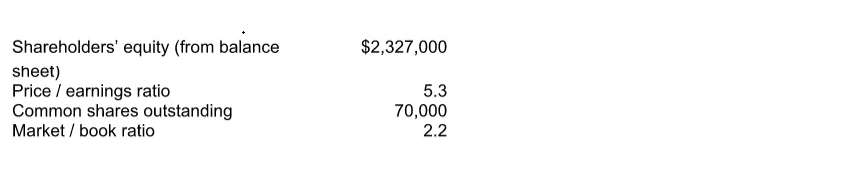# Problem: You are given the following information about a company: Calculate the price of a share of the company’s common stock. a. $176.19 b.$13.80 c. $96.76 d.$80.09 e. $73.13 ###### FREE Expert Solution ###### Problem Details You are given the following information about a company: Calculate the price of a share of the company’s common stock. a.$176.19

b. $13.80 c.$96.76

d. $80.09 e.$73.13Frequently Asked Questions

What scientific concept do you need to know in order to solve this problem?

Our tutors have indicated that to solve this problem you will need to apply the Ratios: Price-Earnings Ratio (PE Ratio) concept. You can view video lessons to learn Ratios: Price-Earnings Ratio (PE Ratio). Or if you need more Ratios: Price-Earnings Ratio (PE Ratio) practice, you can also practice Ratios: Price-Earnings Ratio (PE Ratio) practice problems.

What is the difficulty of this problem?

Our tutors rated the difficulty ofYou are given the following information about a company: Ca...as medium difficulty.